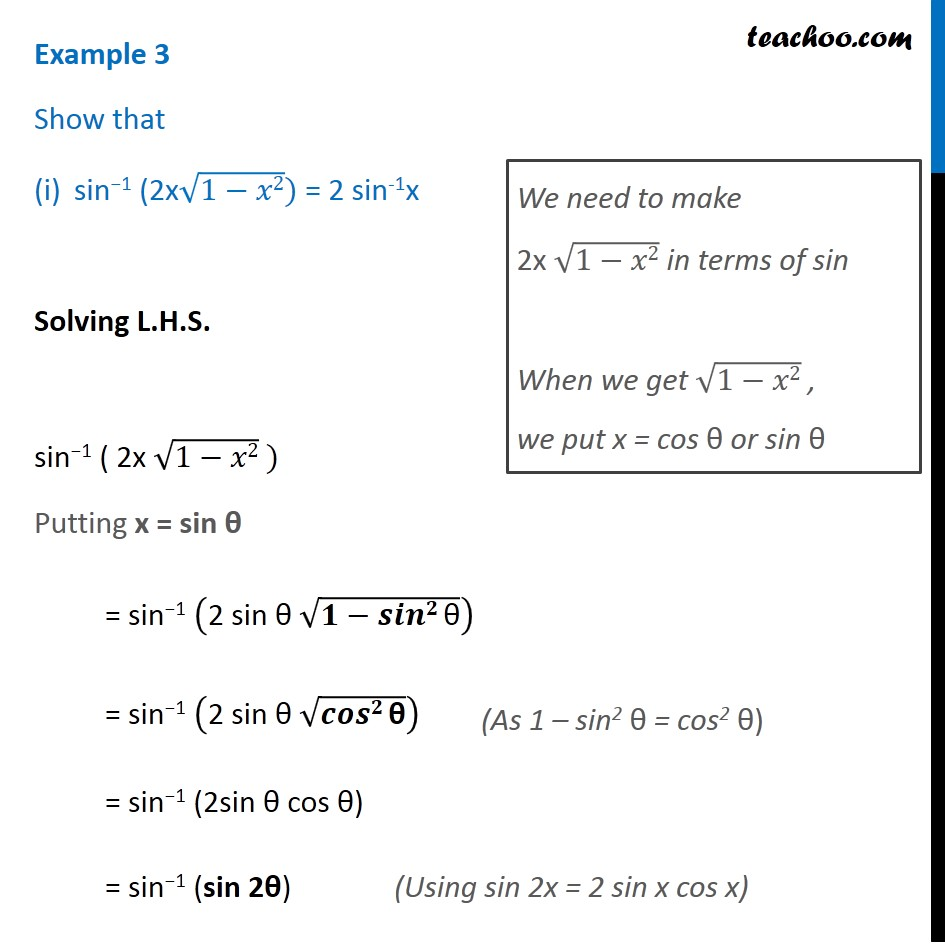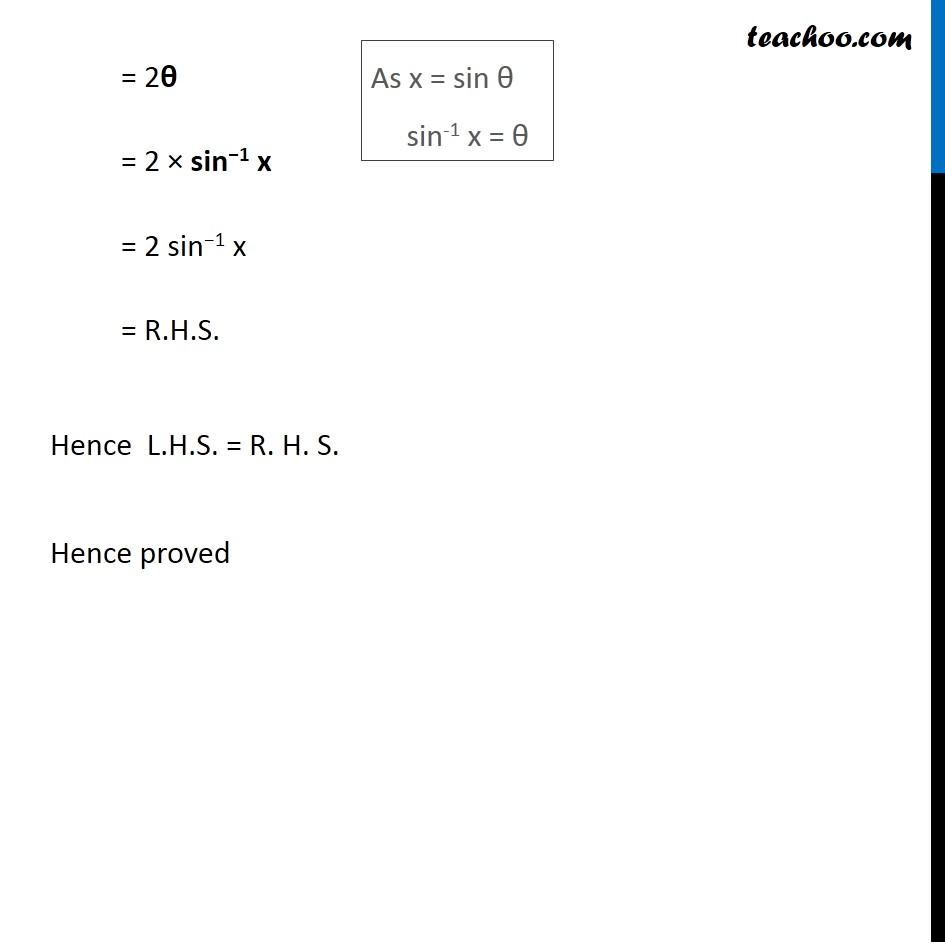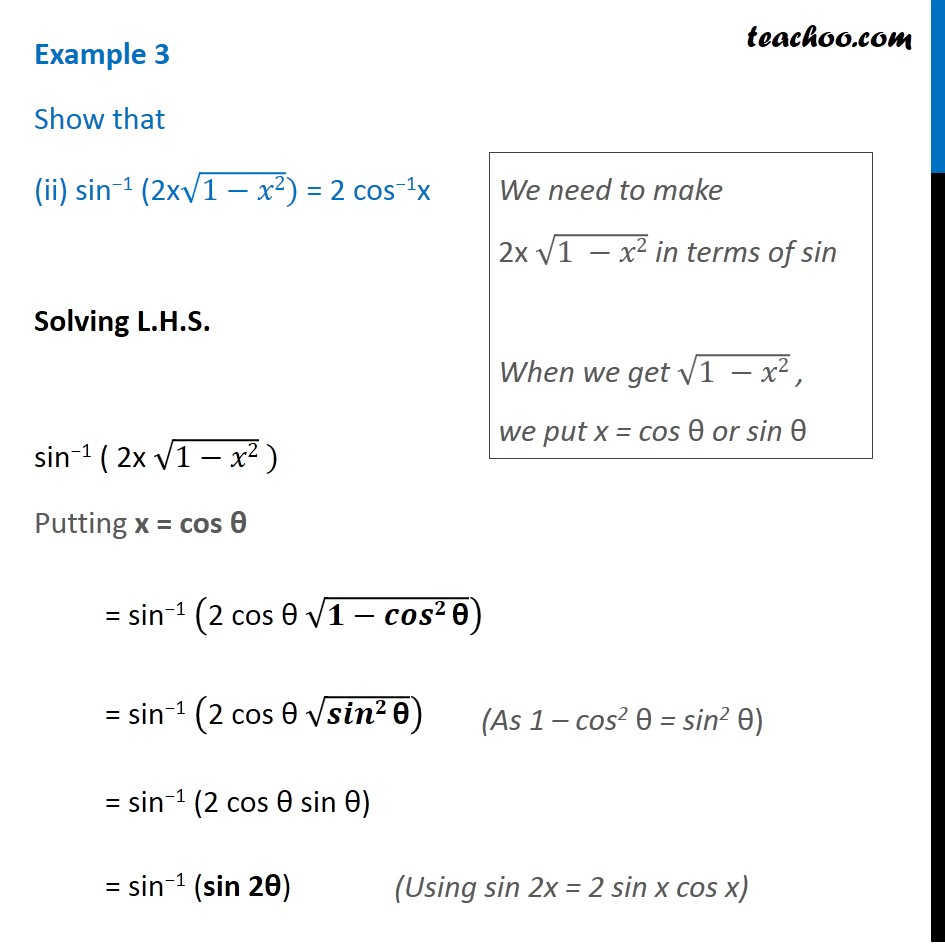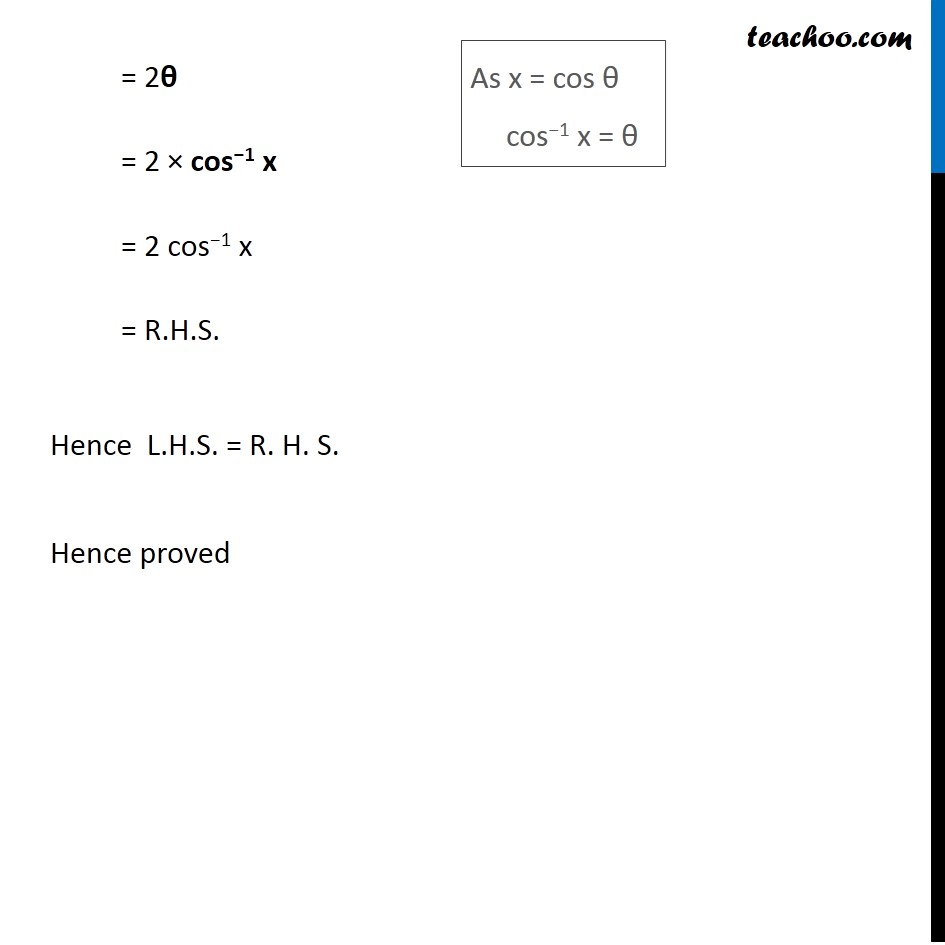Subscribe to our Youtube Channel - https://you.tube/teachoo

1. Chapter 2 Class 12 Inverse Trigonometric Functions
2. Serial order wise
3. Examples

Transcript

Example 3 Show that sin−1 (2x√(1−𝑥2)) = 2 sin-1x Solving L.H.S. sin−1 ( 2x √(1−𝑥2) ) Putting x = sin θ = sin−1 ("2 sin θ " √(𝟏−𝒔𝒊𝒏𝟐" θ" )) = sin−1 ("2 sin θ " √(𝒄𝒐𝒔𝟐" θ" )) = sin−1 (2sin θ cos θ) = sin−1 (sin 2θ) We need to make 2x √(1−𝑥2) in terms of sin When we get √(1−𝑥2) , we put x = cos θ or sin θ = 2θ = 2 × sin−1 x = 2 sin−1 x = R.H.S. Hence L.H.S. = R. H. S. Hence proved As x = sin θ sin-1 x = θ Example 3 Show that (ii) sin−1 (2x√(1−𝑥2)) = 2 cos−1x Solving L.H.S. sin−1 ( 2x √(1−𝑥2) ) Putting x = cos θ = sin−1 ("2 cos θ " √(𝟏−𝒄𝒐𝒔𝟐" θ" )) = sin−1 ("2 cos θ " √(𝒔𝒊𝒏𝟐" θ" )) = sin−1 (2 cos θ sin θ) = sin−1 (sin 2θ) We need to make 2x √(1 −𝑥2) in terms of sin When we get √(1 −𝑥2) , we put x = cos θ or sin θ (As 1 – cos2 θ = sin2 θ) = 2θ = 2 × cos−1 x = 2 cos−1 x = R.H.S. Hence L.H.S. = R. H. S. Hence proved As x = cos θ cos−1 x = θ

Examples